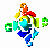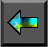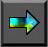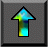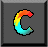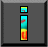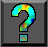Next: 2.4 Agglomeration Up: 2 Designing Parallel Algorithms Previous: 2.2 Partitioning

# 2.3 Communication

The tasks generated by a partition are intended to execute concurrently but cannot, in general, execute independently. The computation to be performed in one task will typically require data associated with another task. Data must then be transferred between tasks so as to allow computation to proceed. This information flow is specified in the communication phase of a design.

The definition of a channel involves an intellectual cost and the sending of a message involves a physical cost. Hence, we avoid introducing unnecessary channels and communication operations. In addition, we seek to optimize performance by distributing communication operations over many tasks and by organizing communication operations in a way that permits concurrent execution.

In domain decomposition problems, communication requirements can be difficult to determine. Recall that this strategy produces tasks by first partitioning data structures into disjoint subsets and then associating with each datum those operations that operate solely on that datum. This part of the design is usually simple. However, some operations that require data from several tasks usually remain. Communication is then required to manage the data transfer necessary for these tasks to proceed. Organizing this communication in an efficient manner can be challenging. Even simple decompositions can have complex communication structures.

In contrast, communication requirements in parallel algorithms obtained by functional decomposition are often straightforward: they correspond to the data flow between tasks. For example, in a climate model broken down by functional decomposition into atmosphere model, ocean model, and so on, the communication requirements will correspond to the interfaces between the component submodels: the atmosphere model will produce values that are used by the ocean model, and so on (Figure 2.3).

In the following discussion, we use a variety of examples to show how communication requirements are identified and how channel structures and communication operations are introduced to satisfy these requirements. For clarity in exposition, we categorize communication   patterns along four loosely orthogonal axes: local/global, structured/unstructured, static/dynamic, and synchronous/asynchronous.

•   In local communication, each task communicates with a small set of other tasks (its ``neighbors''); in contrast, global communication requires each task to communicate with many tasks.
•   In structured communication, a task and its neighbors form a regular structure, such as a tree or grid; in contrast, unstructured communication networks may be arbitrary graphs.
•   In static communication, the identity of communication partners does not change over time; in contrast, the identity of communication   partners in dynamic communication structures may be determined by data computed at runtime and may be highly variable.
•   In synchronous communication, producers and consumers execute in a coordinated fashion, with producer/consumer pairs cooperating in   data transfer operations; in contrast, asynchronous communication may require that a consumer obtain data without the cooperation of the producer.

## 2.3.1 Local Communication

A local communication structure is obtained when an operation requires data from a small number of other tasks. It is then straightforward to define channels that link the task responsible for performing the operation (the consumer) with the tasks holding the required data (the producers) and to introduce appropriate send and receive operations in the producer and consumer tasks, respectively.

For illustrative purposes, we consider the communication requirements associated with a simple numerical computation, namely a Jacobi finite difference method. In this class of numerical method, a multidimensional grid is repeatedly updated by replacing the value at each point with some function of the values at a small, fixed number of neighboring points. The set of values required to update a single   grid point is called that grid point's stencil. For example, the following expression uses a five-point stencil to update each element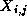of a two-dimensional grid X :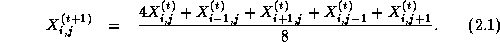This update is applied repeatedly to compute a sequence of values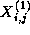,, and so on. The notation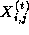denotes the value of grid pointat step t .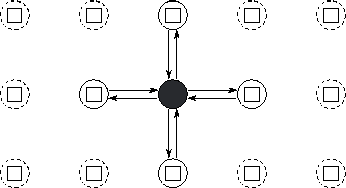Figure 2.4: Task and channel structure for a two-dimensional finite difference computation with five-point update stencil. In this simple fine-grained formulation, each task encapsulates a single element of a two-dimensional grid and must both send its value to four neighbors and receive values from four neighbors. Only the channels used by the shaded task are shown.

Let us assume that a partition has used domain decomposition techniques to create a distinct task for each point in the two-dimensional grid. Hence, a task allocated the grid pointmust compute the sequence

```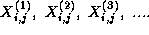```
This computation requires in turn the four corresponding sequences
```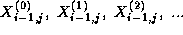```
```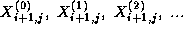```
```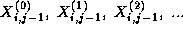```
```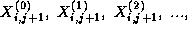```
which are produced by the four tasks handling grid points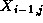,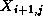,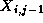, and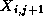, that is, by its four neighbors in the grid. For these values to be communicated, we define channels linking each task that requires a value with the task that generates that value. This yields the channel structure illustrated in Figure 2.4. Each task then executes the following logic:

```
for  t=0
to  T-1

sendto each neighbor

receive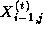,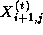,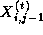,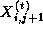from neighbors

compute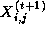using Equation 2.1

endfor

```

We observed earlier that the best sequential and parallel solutions to a problem may use different techniques. This situation arises in finite difference problems. In sequential computing,   Gauss-Seidel update strategies are often preferred over Jacobi strategies because they allow solutions of comparable accuracy to be obtained using fewer iterations. In a Gauss-Seidel scheme, elements are updated in a particular order so that the computation of each element can use the most up-to-date value of other elements. For   example, the Jacobi update of Equation 2.1 may be reformulated as follows (notice the use of values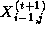and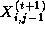):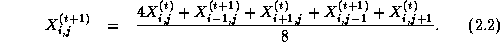While Jacobi schemes are trivial to parallelize (all grid points can be updated concurrently), this is not the case for all Gauss-Seidel   schemes. For example, the update scheme of Equation 2.2 allows only an average of around N/2 points within an N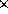N grid to be updated concurrently. Fortunately, many different Gauss-Seidel orderings are possible for most problems, and we are usually free to choose the ordering that maximizes available parallelism. In particular, we can choose to update first the odd-numbered elements and then the even-numbered elements of an array. Each update uses the most recent information, yet the updates to the odd-numbered points are independent and can proceed concurrently, as can the updates to the even-numbered points.   This update strategy yields what is referred to as a red-black algorithm, since the points can be thought of as being colored as on a chess board: either red (odd) or black (even); points of the same color can be updated concurrently. Figure 2.5 illustrates both the Gauss-Seidel scheme of Equation 2.2 and a red-black scheme, and shows how the latter scheme increases opportunities for parallel execution.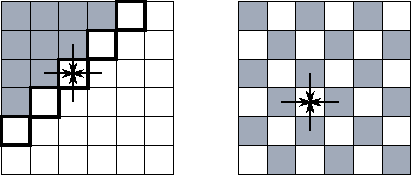Figure 2.5: Two finite difference update strategies, here applied on a two-dimensional grid with a five-point stencil. In both figures, shaded grid points have already been updated to step t+1 ; unshaded grid points are still at step t . The arrows show data dependencies for one of the latter points. The figure on the left illustrates a simple Gauss-Seidel scheme and highlights the five grid points that can be updated at a particular point in time. In this scheme, the update proceeds in a wavefront from the top left corner to the bottom right. On the right, we show a red-black update scheme. Here, all the grid points at step t can be updated concurrently.

This example indicates the important role that choice of solution strategy can play in determining the performance of a parallel program. While the simple Gauss-Seidel update strategy of Equation 2.2 may be appropriate in a sequential program, it is not ideal on a parallel computer. The Jacobi update strategy is efficient on a parallel computer but is inferior numerically. The red-black scheme combines the advantages of both approaches.

## 2.3.2 Global Communication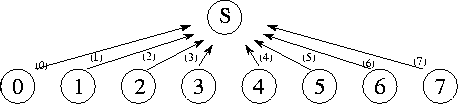Figure 2.6: A centralized summation algorithm that uses a central manager task (S) to sum N numbers distributed among N tasks. Here, N=8 , and each of the 8 channels is labeled with the number of the step in which they are used.

A global communication operation is one in which many tasks must participate. When such operations are implemented, it may not be sufficient simply to identify individual producer/consumer pairs. Such an approach may result in too many communications or may restrict opportunities for concurrent execution. For example, consider the   problem of performing a parallel reduction operation, that   is, an operation that reduces N values distributed over N tasks using a commutative associative operator such as addition: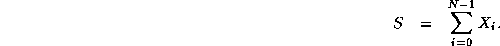Let us assume that a single ``manager'' task requires the result S of this operation. Taking a purely local view of communication, we recognize that the manager requires values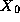,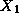, etc., from tasks 0, 1, etc. Hence, we could define a communication structure that allows each task to communicate its value to the manager independently. The manager would then receive the values and add them into an accumulator (Figure 2.6). However, because the manager can receive and sum only one number at a time, this approach takes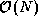time to sum N numbers---not a very good parallel algorithm!

This example illustrates two general problems that can hinder efficient parallel execution in algorithms based on a purely local view of communication:

1. The algorithm is centralized : it does not distribute computation and communication. A single task (in this case, the manager task) must participate in every operation.
2. The algorithm is sequential : it does not allow multiple computation and communication operations to proceed concurrently.
We must address both these problems to develop a good parallel algorithm.

#### Distributing Communication and Computation.

We first consider the problem of distributing the computation and communication associated with the summation. We can distribute the summation of the N numbers by making each task i , 0<i<N-1 , compute the sum: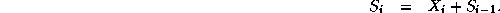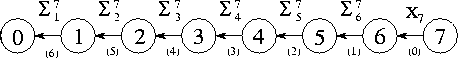Figure 2.7: A summation algorithm that connects N tasks in an array in order to sum N numbers distributed among these tasks. Each channel is labeled with the number of the step in which it is used and the value that is communicated on it.

The communication requirements associated with this algorithm can be satisfied by connecting the N tasks in a one-dimensional array (Figure 2.7). Task N-1 sends its value to its neighbor in this array. Tasks 1 through N-2 each wait to receive a partial sum from their right-hand neighbor, add this to their local value, and send the result to their left-hand neighbor. Task 0 receives a partial sum and adds this to its local value to obtain the complete sum. This algorithm distributes the N-1 communications and additions, but permits concurrent execution only if multiple summation operations are to be performed. (The array of tasks can then be used as a pipeline, through which flow partial sums.) A single summation still takes N-1 steps.

#### Uncovering Concurrency: Divide and Conquer.

Opportunities for concurrent computation and communication can often be uncovered by applying a problem-solving strategy called divide and conquer. To solve a complex problem (such as summing N numbers), we seek to partition it into two or more simpler problems of roughly equivalent size (e.g., summing N/2 numbers). This process is applied recursively to produce a set of subproblems that cannot be subdivided further (e.g., summing two numbers). The strategy is summarized in Algorithm 2.1. The divide-and-conquer technique is effective in parallel computing when the subproblems generated by problem partitioning can be solved concurrently. For example, in the summation problem, we can take   advantage of the following identity (, n an integer):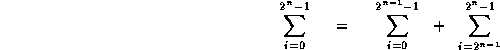The two summations on the right hand side can be performed concurrently. They can also be further decomposed if n>1 , to give the tree structure illustrated in Figure 2.8. Summations at the same level in this tree of height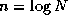can be performed concurrently, so the complete summation can be achieved in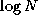rather than N steps.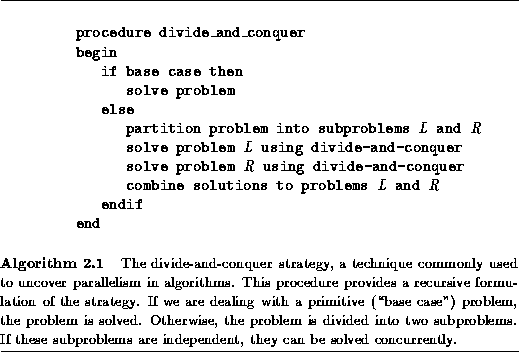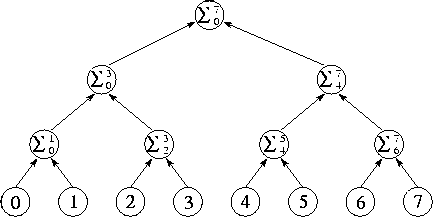Figure 2.8: Tree structure for divide-and-conquer summation algorithm with N=8 . The N numbers located in the tasks at the bottom of the diagram are communicated to the tasks in the row immediately above; these each perform an addition and then forward the result to the next level. The complete sum is available at the root of the tree aftersteps.

In summary, we observe that in developing an efficient parallel summation algorithm, we have distributed the N-1 communication and computation operations required to perform the summation and have modified the order in which these operations are performed so that they can proceed concurrently. The result is a regular communication structure in which each task communicates with a small set of neighbors.

## 2.3.3 Unstructured and Dynamic Communication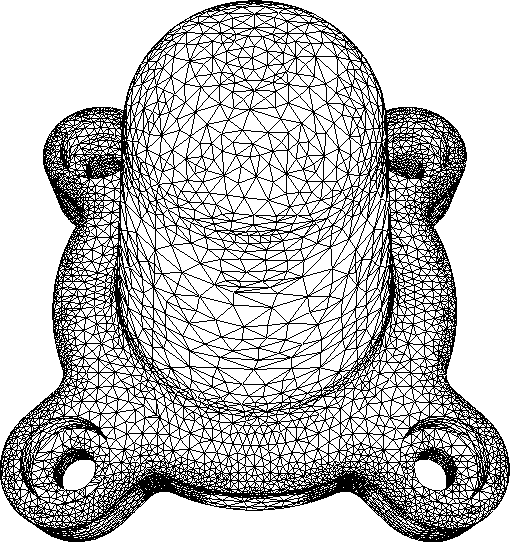Figure 2.9: Example of a problem requiring unstructured communication. In this finite element mesh generated for an assembly part, each vertex is a grid point. An edge connecting two vertices represents a data dependency that will require communication if the vertices are located in different tasks. Notice that different vertices have varying numbers of neighbors. (Image courtesy of M. S. Shephard.)

The examples considered previously are all of static, structured communication, in which a task's communication partners form a regular pattern such as a tree or a grid and do not change over time. In other cases, communication patterns may be considerably more   complex. For example, in finite element methods used in engineering   calculations, the computational grid may be shaped to follow an irregular object or to provide high resolution in critical regions (Figure 2.9). Here, the channel structure representing the communication partners of each grid point is quite irregular and data-dependent and, furthermore, may change over time if the grid is refined as a simulation evolves.

Unstructured communication patterns do not generally cause conceptual difficulties in the early stages of a design. For example, it is straightforward to define a single task for each vertex in a finite element graph and to require communication for each edge. However, unstructured communication complicates the tasks of agglomeration and mapping. In particular, sophisticated algorithms can be required to determine an agglomeration strategy that both creates tasks of approximately equal size and minimizes communication requirements by creating the least number of intertask edges. Algorithms of this sort are discussed in Section 2.5.1. If communication   requirements are dynamic, these algorithms must be applied frequently during program execution, and the cost of these algorithms must be weighed against their benefits.

## 2.3.4 Asynchronous Communication

The examples considered in the preceding section have all featured synchronous communication, in which both producers and consumers are aware when communication operations are required, and producers explicitly send   data to consumers. In asynchronous communication, tasks that   possess data (producers) are not able to determine when other tasks (consumers) may require data; hence, consumers must explicitly request data from producers.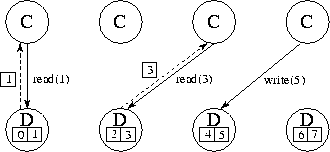Figure 2.10: Using separate ``data tasks'' to service read and write requests on a distributed data structure. In this figure, four computation tasks (C) generate read and write requests to eight data items distributed among four data tasks (D). Solid lines represent requests; dashed lines represent replies. One compute task and one data task could be placed on each of four processors so as to distribute computation and data equitably.

This situation commonly occurs when a computation is structured as a set of tasks that must periodically read and/or write elements of a shared data structure. Let us assume that this data structure is too large or too frequently accessed to be encapsulated in a single task. Hence, a mechanism is needed that allows this data structure to be distributed while supporting asynchronous read and write operations on its components. Possible mechanisms include the following.

1.   The data structure is distributed among the computational tasks. Each   task both performs computation and generates requests for data located in other tasks. It also periodically interrupts its own computation and polls for pending requests.

2. The distributed data structure is encapsulated in a second set of tasks responsible only for responding to read and write requests (Figure 2.10).

3. On a computer that supports a shared-memory programming model, computational tasks can access shared data without any special arrangements. However, care must be taken to ensure that read and write operations on this shared data occur in the proper order.

Each strategy has advantages and disadvantages; in addition, the performance characteristics of each approach vary from machine to machine. The first strategy can result in convoluted, nonmodular programs because of the need to intersperse polling operations   throughout application code. In addition, polling can be an expensive operation on some computers, in which case we must trade off the cost of frequent polling against the benefit of rapid response to remote requests. The second strategy is more modular: responsibility for the shared data structure is encapsulated in a separate set of tasks. However, this strategy makes it hard to exploit locality because, strictly speaking, there are no local data: all read and write operations require communication. Also, switching between the computation and data tasks can be expensive on some machines.

## 2.3.5 Communication Design Checklist

Having devised a partition and a communication structure for our parallel algorithm, we now evaluate our design using the following design checklist. As in Section 2.2.3, these are guidelines intended to identify nonscalable features, rather than hard and fast rules. However, we should be aware of when a design violates them and why.

1. Do all tasks perform about the same number of communication operations? Unbalanced communication requirements suggest a nonscalable construct. Revisit your design to see whether communication operations can be distributed more equitably. For example, if a frequently accessed data structure is encapsulated in a single task, consider distributing or replicating this data structure.

2. Does each task communicate only with a small number of neighbors? If each task must communicate with many other tasks, evaluate the possibility of formulating this global communication in terms of a local communication structure, as was done in the pairwise interactions algorithm of Section 1.4.2 and the summation algorithm of Section 2.3.2.

3. Are communication operations able to proceed concurrently? If not, your algorithm is likely to be inefficient and nonscalable. Try to use divide-and-conquer techniques to uncover concurrency, as in the summation algorithm of Section 2.3.2.

4. Is the computation associated with different tasks able to proceed concurrently? If not, your algorithm is likely to be inefficient and nonscalable. Consider whether you can reorder communication and computation operations. You may also wish to revisit your problem specification, as was done in moving from a simple Gauss-Seidel to a red-black algorithm in Section 2.3.1.Next: 2.4 Agglomeration Up: 2 Designing Parallel Algorithms Previous: 2.2 Partitioning